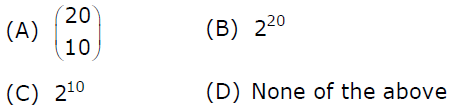# GATE | GATE-CS-2007 | Question 84

Suppose that a robot is placed on the Cartesian plane. At each step it is allowed to move either one unit up or one unit right, i.e., if it is at (i,j) then it can move to either (i+1,j) or (i,j+1).
How many distinct paths are there for the robot to reach the point (10,10) starting from the initial position (0, 0)(A) A
(B) B
(C) C
(D) D

Explanation: At each move, robot can move either 1 unit right, or 1 unit up, and there will be 20 such moves required to reach (10,10) from (0,0). So we have to divide these 20 moves, numbered from 1 to 20, into 2 groups: right group and up group.

Right group contains those moves in which we move right, and up group contains those moves in which we move up.

Each group contains 10 elements each. So basically, we have to divide 20 things into 2 groups of 10 10 things each, i.e., we need to find all possible arrangements of {r, r, r, r, r, r, r, r, r, r, u, u, u, u, u, u, u, u, u, u} where r represents right move and u represents up move. The arrangements can can be done in 20! / (10!∗10!) = 20C10 ways. So option (A) is correct.

My Personal Notes arrow_drop_up
Article Tags :

Be the First to upvote.

Please write to us at contribute@geeksforgeeks.org to report any issue with the above content.